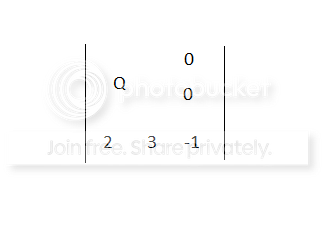# Submatrix and element alignment within matrix

I'm having trouble aligning submatrices and elements within the same matrix. Essentially, what I want to do is this (excuse the terrible paint-job):Where the submatrix Q is located at the middle of the upper-left four matrix cells. I'm primarily familiar with the array environment: is this a possibility there?

How about a matrix inside a matrix?

\documentclass{article}
\pagestyle{empty}% for cropping
\usepackage{amsmath}
\begin{document}
$\begin{vmatrix} Q & \begin{matrix} 0 \\ 0 \end{matrix} \\ \begin{matrix} 2 & 3 \end{matrix} & -1 \end{vmatrix}$
\end{document}Or

\documentclass{article}
\pagestyle{empty} % for cropping
\usepackage{amsmath}
\begin{document}
$\left| \begin{array}{cr} Q & \begin{matrix} 0 \\ 0 \end{matrix} \\ \begin{matrix} 2 & 3 \end{matrix} & -1 \end{array} \right|$
\end{document}


using the array environment that allows a better alignment.or maybe something like

\documentclass{article}
\pagestyle{empty} % for cropping
\usepackage{amsmath}
\usepackage{arydshln}
\begin{document}
$\left| \begin{array}{c;{2pt/2pt}r} \mbox{\LARGE Q} & \begin{matrix} 0 \\ 0 \end{matrix} \\ \hdashline[2pt/2pt] \begin{matrix} 2 & 3 \end{matrix} & -1 \end{array} \right|$
\end{document}


that modifies the size of Q and includes dashed lines between columns using the arydshln package.• amsmath doesn't load the array package, and the array as well as the matrix environment are defined in plain LaTeX so need for amsmath in your example. Jun 29, 2014 at 17:17
• @Henri is correct, excuse me, but if i delete (or comment) the amsmath package i get an error (about matrix?). i already fixed my answer. Jun 29, 2014 at 17:32
• You're right. Seems like the standard matrix doesn't like to be nested, hence you will still have to use the amsmath package. Jun 29, 2014 at 18:03
• in cases like this, the "sub"element (here "Q") is often set in a larger size. Jun 29, 2014 at 22:02
• @skpblack -- i think it may be one size larger than necessary, but yes, that's definitely what i had in mind. Jun 30, 2014 at 2:29

A third solution with \multicolumn and multirow. I defined a \block macro with two arguments: the number of rows and columns that the block takes up (expressed as a pair) and what it puts in the block:

\documentclass{article}
\usepackage[utf8]{inputenc}
\usepackage{fourier, heuristica}
\usepackage{multirow}
\usepackage{mathtools}
\def\block(#1,#2)#3{\multicolumn{#2}{c}{\multirow{#1}{*}{$#3$}}}

\begin{document}

$\begin{vmatrix*}[r] \block(2,2){Q} & 0 \\ & & 0 \\ 2 & 3 & \mathllap{-}1 \end{vmatrix*}$

$\begin{vmatrix*}[r] 2 & 3 & -1 & 0 \\ 0 & \block(2,2){P} & 0 \\ 4 & & & 0 \\ 3 &2 & 1 & 2 \end{vmatrix*}$

\end{document}• @Svend Tveskæg: Sorry, I mixed up with my clipboard manager. Fix this at once. Jun 29, 2014 at 22:22

With {vNiceMatrix} of nicematrix.

\documentclass{article}
\usepackage{nicematrix}

\begin{document}

$\begin{vNiceMatrix} \Block{2-2}<\Large>{Q} & & 0 \\ & & 0 \\ 2 & 3 & -1 \end{vNiceMatrix}$

\end{document}